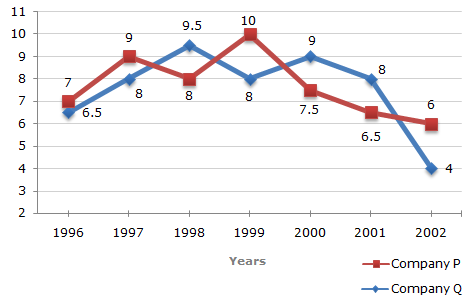# Data Interpretation - Line Charts - Discussion

### Discussion :: Line Charts - Line Chart 8 (Q.No.3)

Two different finance companies declare fixed annual rate of interest on the amounts invested with them by investors. The rate of interest offered by these companies may differ from year to year depending on the variation in the economy of the country and the banks rate of interest. The annual rate of interest offered by the two Companies P and Q over the years are shown by the line graph provided below.

Annual Rate of Interest Offered by Two Finance Companies Over the Years.3.

In 2000, a part of Rs. 30 lakhs was invested in Company P and the rest was invested in Company Q for one year. The total interest received was Rs. 2.43 lakhs. What was the amount invested in Company P?

 [A]. Rs. 9 lakhs [B]. Rs. 11 lakhs [C]. Rs. 12 lakhs [D]. Rs. 18 lakhs

Explanation:

Let Rs. x lakhs be invested in Company P in 2000, the amount invested in Company Q in 2000 = Rs. (30 - x) lakhs.

Total interest received from the two Companies after 1 year

= Rs. [(7.5% of x) + {9% of (30 - x)}] lakhs

 = Rs.2.7 -1.5xlakhs. 1002.7 -1.5x= 2.43x = 18. 100

 Bhavin Shah said: (Jul 20, 2011) Can you explain the steps in detail How did 1.5x came and 2.7 also????

 Vasanth said: (Aug 6, 2011) [(7.5% of x)+ ( 9% of (30-x))] of this take (9% of (30-x))= (9% of 30) - (9% of x) and (9% of 30)= 27/100 =2.7 and as whole [(7.5% of x)+ 2.7 - (9% of x)] =2.7 - (1.5% of x) =2.7 - (1.5x/100)

 Dina said: (Sep 26, 2012) More detailed steps:. (7.5% of x) + ( (9% of (30-x) ) = 2.43. 0.075x + 0.09 (30-x) = 2.43. 0.075x + 2.7 - 0.09x = 2.43 (subtract all the x s together). -0.015x + 2.7 = 2.43. -0.015x = 2.43 -2.7 (2.7 will go to the other side). -0.015x = - 0.27 (both negative signs will cross each other). x= 0.27/0.015 (both negative signs will cross each other). x= 18.

 Naveen said: (Aug 19, 2014) Why we take 7.5 of x and 9%of 30-x?

 Vishwanath said: (Sep 30, 2014) Because 7.5 is the interest rate of company P in the year 2000 and Q as well.

 Lovely said: (Mar 10, 2015) Is there any short cut to solve these types of questions?

 Tushar Cool said: (Apr 6, 2015) For 30 lakhs he receives interest @ 9% it amounts to 2.7 l and @ 7.5% it amounts to 2.25 l actually he received 2.43 l. So 2.7-2.43 = 0.27. And 2.43-2.25 = 0.18. The ratio becomes 0.27:0.18. i.e. 3:2 so amount deposited in higher ROI will be 12 and lower ROI will be 18 (30 lakh in ratio of 3:2). Do it like mixture and allegations?

 Mizewar said: (Feb 18, 2016) It does not make sense for me! Can you please explain with the very small steps?

 Kiran said: (Mar 6, 2017) @Tushar. 2.7 - 2.43 Please explain this step.

 Ravi said: (Jul 14, 2017) Good explanation, thanks @Tushar.

 Sreeraj said: (Aug 7, 2020) How we got the rate as 7.5 and 9%?

 Talop Thazey said: (Oct 27, 2020) Let amt invested in P = x. And Q = (30-x). (P) 7.5% of x +( Q) 9%of ( 30-x ). Sol. 7.5/100*x + 9/100*30 - 9/100*x Take that 7.5 to right side and subtract with 9 We will get 1.5x/100 9/100 * 30 = 2.70, 2.70 - (1.5x/100) = 2.43, 1.5x/100 = 2.43-2.70, 1.5/100. = 0.27, 0.27 * 100/1.5x, 270/1.5x, X = 18.